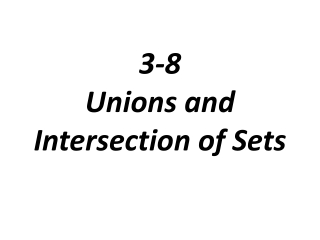Download Presentation3-8 Unions and Intersection of Sets

# 3-8 Unions and Intersection of Sets

Télécharger la présentation## 3-8 Unions and Intersection of Sets

- - - - - - - - - - - - - - - - - - - - - - - - - - - E N D - - - - - - - - - - - - - - - - - - - - - - - - - - -
##### Presentation Transcript

1. 3-8 Unions and Intersection of Sets

2. The Union of two or more sets is the set that contains all elements of the sets Symbol: How to find: list the elements that are in either set, or in both sets

3. Problem 1: Union of Sets In your left pocket, you have a quarter, a paper clip, and a key. In your right pocket, you have a penny, a quarter, a pencil, and a marble. What is a set that represents the different items in your pockets

4. P={0, 1, 2, 3, 4} Q={2, 4} What is

5. The Intersection of two or more sets is the set of elements that are common to every set Symbol: How to Find: list only the elements that are in both sets

6. Disjoint sets have no elements in common The intersection of disjoint sets is the empty set

7. Problem 2: Intersection of Sets X={x|x is a natural number less than 19} Y={y|y is an odd integer} Z={z|z is a multiple of 6} What is ?

8. X={x|x is a natural number less than 19} Y={y|y is an odd integer} Z={z|z is a multiple of 6} What is ?

9. Problem 3: Making a Venn Diagram A={x|x is one of the first five letters in the English alphabet} B={x|x is a vowel} C={x|x is a letter in the word VEGETABLE} Which letters are in all three sets (Draw a Venn Diagram)?

10. Problem 4: Using a Venn Diagram to Show Numbers of Elements Of 500 Commuters polled, some drive to work, some take public transportation, and some do both. Two hundred commuters drive to work and 125 use both types of transportation. How many commuters take public transportation?